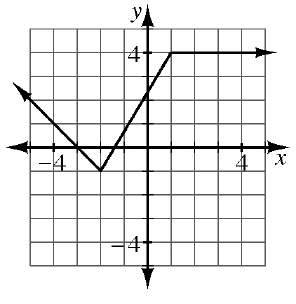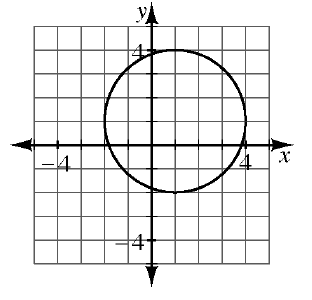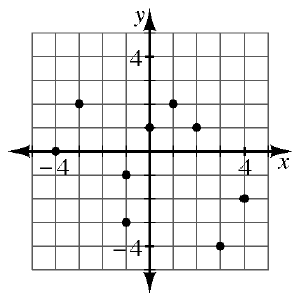### Home > CC4 > Chapter 8 > Lesson 8.1.5 > Problem8-76

8-76.

The graphs of several relations are shown below. Decide if each is a function. If the relation is not a function, explain why not. Homework Help ✎

1.A relation is only a function if there is only one $y$-value for each $x$-value. Are there any $x$-values on this graph that have multiple $y$-values?

This relation is a function.

1.See the help for part (a).

This relation is not a function. Be sure to explain why.

1.See the help for part (a).